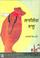### Fundamentals of Electrical Engineering

#### Course Description

The overview of development and current trends in electrical engineering and computer science. Fundamentals of electricity, capacitance. Electric current and electrical phenomena. Fundamentals of magnetism, inductance and mutual inductance. Concepts, elements and topology of electric circuits. Kirchhoff's laws. Elementary DC circuits. Circuits with capacitors. Complex DC circuits (bridge circuit, star-delta transformation, circuits with multiple sources). Superposition, Thevenin's, Norton's and Millman's theorem. Current and voltage waveforms. Complex calculus in analysis of AC circuits. RLC circuits. Topographic and locus diagrams. Frequency characteristics. Instantaneous, real, reactive and apparent power. AC circuits with multiple sources. Polyphase system. Harmonic analysis, applications in circuit analysis. Transients.

#### General Competencies

The course gives understanding of concepts, laws and principles regarding electrical circuits. After finishing this course students will be able to analyze DC and AC electrical circuits and understand underlying physical phenomena.

#### Learning Outcomes

1. define and understand the fundamental concepts related to electricity, magnetism and electric circuit theory.
2. understand and apply Kirchhoff's Laws to DC and AC circuit analysis.
3. understand and apply phasors for sinusoidal steady-state AC circuit analysis.
4. analyze DC and AC circuits by following circuit analysis methods and theorems: nodal analysis, star-delta transformation, transformation between real source models, Millman's, Thévenin's and Norton's theorems.
5. understand and apply the principle of linearity and superposition to AC and DC circuits.
6. analyze circuits with non-sinusoidal sources by harmonic analysis.
7. analyze transient response of first order circuits (series RC and RL).
8. use basic laboratory measurement equipment including the power supplies, ammeters, voltmeters, ohmmeters, digital multimeters, function generators, and oscilloscopes as well as to conduct experiments, to measure basic quantities in electric circuits, and to interpret data.

#### Forms of Teaching

Lectures

Involvement in lectures

Exams

Computer aided, written, amd oral exams

Laboratory Work

Laboratory work

Consultations

Lecturers consultations

Other

Individual work and learning

E-learning

Homework

Continuous Assessment Exam
Laboratory Exercises 0 % 15 % 0 % 15 %
Homeworks 0 % 5 % 0 % 5 %
Class participation 0 % 4 % 0 % 4 %
Mid Term Exam: Written 0 % 26 % 0 %
Final Exam: Written 0 % 26 %
Final Exam: Oral 24 %
Exam: Written 0 % 52 %
Exam: Oral 24 %

#### Week by Week Schedule

1. Course description with the overview of development and current trends in electrical engineering and computer science. Fundamentals of electricity, (atoms and their structure, electric charge, electric force, electric field, electric potential, capacitance and capacitor, capacitor energy.
2. Electric current and electrical phenomena (resistance, Ohm's and Joule's Law, electrical energy and power). Concepts, elements and topology of electric circuits. Kirchhoff's laws.
3. Elementary DC circuits. Circuits with capacitors.
4. More complex DC circuits (bridge circuit, star-delta transformation, circuits with multiple sources). Superposition principle.
5. Fundamentals of magnetism (magnetic force, magnetic field, Faraday's Law, inductance and mutual inductance, inductor energy).
6. Current and voltage waveforms. Complex calculus in analysis of AC circuits. Phasors.
7. RLC circuits. Frequency characteristics.
8. Midterm exam.
9. Power and energy in AC circuits.
10. Topographic and locus diagrams. Complex AC circuits.
11. Circuit analysis methods.
12. Poliphase systems.
13. Transients. Characteristics of nonsinusoidal periodical waveforms.
14. Waveform harmonic analysis.
15. Final exam.

#### Study Programmes

[FER2-HR] Electrical Engineering and Information Technology and Computing - study
(1. semester)

#### LiteratureV. Pinter (1989.), Osnove elektrotehnike, I i II dio, Tehnička knjiga, ZagrebE. Šehović, M. Tkalić, I Felja (1992.), Osnove elektrotehnike - zbirka primjera, I dio, Školska knjiga, Zagreb
A. Pavić, I. Felja (1996.), Osnove elektrotehnike 1, auditorne vježbe, Korijandol

#### General

ID 86494
Winter semester
7 ECTS
L0 English Level
L1 e-Learning
90 Lectures
0 Seminar
0 Exercises
15 Laboratory exercises
0 Project laboratory